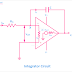When the feedback element of an opamp inverter circuit is replaced by a capacitor then it is called Integrator Circuit or OpAmp Integrator Circuit. We know that the operational amplifier is used as a feedback amplifier and as a feedback amplifier the Opamp performs so many functions such as differentiator, adder, subtractor, etc. In these cases, a resistor is used as the feedback element. We also know that resistor is a resistive element. But when a reactive element or capacitor is used as the feedback element of the operational amplifier circuit, then it provides integrated functions.

Using a Capacitor as a feedback element created an RC network. And now the Opamp produces an output voltage that is proportional to the integral of the input voltage. And this circuit can be used for the mathematical operation of integration with respect to time. Here, the RC network creates a negative feedback current. And this negative current that is generated due to the increase of output voltage will flow through the capacitor.

## Integrator Circuit Diagram

Here, you can see the circuit diagram of Integrator using OpAmp or an operational amplifier.

You can see the resistor Rin and Capacitor C creates an RC network. The RC network is connected to the inverting terminal of the OpAmp. The capacitor is connected between the output and inverting input terminal. the value of the resistor and capacitor is a very important factor. The RC time constant depends upon the value of these elements that control the charging-discharging of the capacitor. Remember that the higher capacitor value will generate the lower output voltage for a particular feedback current.

## Working Principle of Integrator Circuit

First, when a pulse voltage or step voltage is applied to the circuit the capacitor act as a short circuit as it is in uncharged condition. And the capacitor starts charging. So at the initial point, the whole voltage is applied across the capacitor and there is no voltage applied to the input of the OpAmp. The capacitor will be charging at the rate of the time constant set by the RC network. And this charging time the negative of the system will force the Opamp to create an output voltage to maintain a earth or zero potential with respect to the OpAmp inverting terminal.

At the initial point, the voltage across the capacitor will increase linearly until the capacitor is fully charged. The rate at which the voltage increases depend upon the value of the capacitor and resistor. So if a square wave or pulse voltage is applied to the circuit, the output will be a sawtooth waveform. As the resistor and capacitor are connected to a virtual ground, the input does not vary with the charging or discharging of the capacitor. This helps to achieve a liner integration function.

## Application and Uses of Integrator Circuit

1. Integrator Circuits are used in Analog Computers. In older days analog computers are so much used. Analog computers are not so much fast in opration as a digital computer. Even they can function few operations only.

2. These are also used in Analog to Digital Converter Circuit. In short, it is named as ADC. It takes analog signal as input and generates digital signal as output.

3. These are also used for the conversion of analog signals such as modulations.

4. These are also used in Wave Shaping Circuits.

What is Integrator Circuit? Diagram, Working Principle, UseReviewed by Author on November 27, 2021 Rating: 5